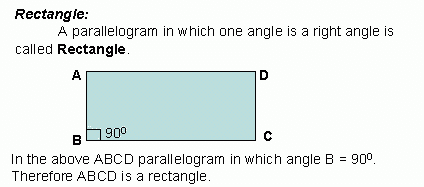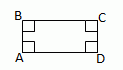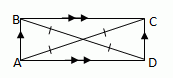Email us to get an instant 20% discount on highly effective K-12 Math & English kwizNET Programs!

#### Online Quiz (WorksheetABCD)

Questions Per Quiz = 2 4 6 8 10

### Geometry3.7 RectangleProperties of Rectangle: Opposite sides of a rectangle are equal or congruent. Opposite angles of a rectangle are equal. All its angles are right angles; or The diagonals of a rectangle bisect each other. Each diagonal divides the rectangle into two congruent triangles. The diagonals of a rectangle are equal. Both pairs of opposite sides are equal and one angle is 90°; or Both pairs of opposite sides are equal and its diagonals are equal. THEOREMS A quadrilateral is a rectangle if and only if it has four right angles.ĐA, ĐB, ĐC, ĐD are 900 A parallelogram is a rectangle, if and only if its diagonals are congruent.AC @ BD Directions: Read the above review points carefully and answer the following questions: Illustrate each of the above review points by drawing a Rectangle. Explain the different properties of a Rectangle, in your own words, with examples. Lilly measures her 10 cm x 6 cm garden plot. She found that all its edges are of angle measure 90°. What will be the shape of her garden? Give reasons. What will be the value of one of the angles of a rectangle if one side is given by 17 cm and other side by 12 cm? Give reasons. If the lengths of the rectangle is x+31 and 5x-9 what is its length. For the following rectangle is the diagonal AC=10, find the mĐDOA, mĐBOC, BO, OD, AO, OC, ACQ 1: "The measure of each angle of a rectangle is 90."TrueFalse Q 2: Find the measure of angle ODA.Answer: Q 3: In a rectangle, one of the diagonals is 5 cm, then the other diagonal will be _______.Data is not sufficient5 cm10 cm Q 4: "A quadrilateral has its sides given by 10 cm, 10 cm, 6 cm and 6 cm. Its diagonals are equal in measure and one of its angles is 90°. Then it is a rectangle."FalseTrue Q 5: One side of a rectangle is given by 10 cm and the other side is given by 6 cm.The values of the remaining two sides will be _______ respectively.10 cm10 cm and 6 cm6 cm Q 6: The diagonals of a Rectangle _______ each other.bisectscoincidesparallels Q 7: The length of the rectangle shown in the figure is ___.156530 Q 8: "The diagonals of a rectangle are given by 6 cm and 5 cm."TrueFalse Question 9: This question is available to subscribers only! Question 10: This question is available to subscribers only!